Monday , May 23 2022# Electricity: 10th CBSE Science Physics

#### Question: What is meant by saying that the potential difference between two points is 1 V?

Answer: As we know that V = W / q

Thus, the potential difference between two points is one volt when one joule of work is done to carry a charge of one coulomb between the two points in the electric field.

#### Question: How much energy is given to one coulomb of charge passing through a 6 V battery?

Answer: Give Q = 1 C, V = 6 V, W = ?
We know that W = QV = 1 × 6 = 6 J

#### Question: On what factors does the resistance of a conductor depend?

Answer: Resistance of a conductor depends upon:
(i) Resistivity of the material.
(ii) Length of the conductor.
(iii) Cross-sectional area of the conductor.

#### Question: Will current flow more easily through a thick wire or thin wire of the same material when connected to the same source? Why?

Answer: The current flows more easily through a thick wire than through a thin wire because the resistance of thick wire is less than that of a thin wire as R ∝ 1/A.

#### Question: Let the resistance of an electrical component remains constant while the potential difference across the two ends of the component decreases to half of its former value. What change will occur in the current through it?

Answer: We know that V ∝ I, therefore, when the potential difference across the two ends of the electrical component becomes half its former value, the current through it also becomes half.

#### Question: Why are the coils of electric toasters and electric irons made of an alloy rather than a pure metal?

Answer: The coils of electric toaster and electric iron are made of an alloy rather than a pure metal because of the following reasons:
(i) The resistivity of an alloy is higher than that of a pure metal.
(ii) It has high melting point and does not oxidize.

#### Question: Use the data in Table 12.2 of NCERT book to answer the following: (a) Which among iron and mercury is a better conductor? (b) Which material is the best conductor? ‘

(a) Iron because its resistivity is less than mercury.
(b) Silver is the best conductor as it has least resistivity.

Page 213

#### Question: Draw a schematic diagram of a circuit consisting of a battery of three cells of 2 V each, a 5 Ω resistor, a 8 Ω resistor and a 12 Ω resistor and a plug key, all connected in series.

Answer: The schematic diagram of the above mentioned circuit is given below: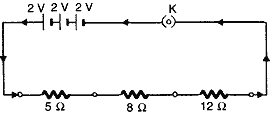#### Question: Redraw the circuit of the above question, putting in an ammeter to measure the current through the resistors and a voltmeter to measure the voltage across the 12 resistor. What would be the reading in the ammeter and the voltmeter?

Answer: The modified circuit is as shown below: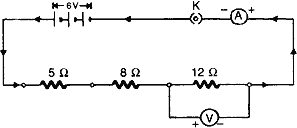Since all the three resistors are connected in series. Therefore, total resistance of the circuit,
R = 5 Ω + 8 Ω + 12 Ω = 25 Ω
Hence, current in the circuit is
I = V/R 6/25 = 0.24 A
This is the reading of the ammeter.
Now, reading of the voltmeter i.e., potential difference across the 12 Ω resistor is
V = IR = 0.24 × 12 = 2.88 V

#### Question: Judge the equivalent resistance when the following are connected in parallel. (a) 1 Ω and 106 Ω (b) 1 Ω , 103 Ω and 106 Ω

Answer: Equivalent resistance in parallel combination of resistors is always less than the least resistance of any resistor in the circuit.

Hence, in both the given cases, the equivalent resistance is less than 1 Ω.

#### Question: An electric lamp of 100 Ω, a toaster of resistance 50 Ω and a water filter of resistance 500 Ω are connected in parallel to a 220 V source. What is the resistance of an electric iron connected to the same source that takes as much current as all three appliances and what is the current flows through it?

Resistance of the electric lamp, R1 = 100 Ω
Resistance of toaster, R2 = 50 Ω
Resistance of water filter, R3 = 500Ω
Since the three appliances are connected in parallel, therefore equivalent resistance is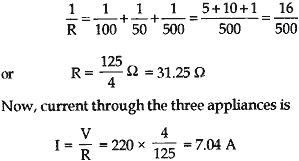Since  the electric iron is connected to the same source (i.e., 220 V) and takes as much current as all three appliances, i.e., I = 7.04 A, therefore, its resistance is equal to R = V/I = 220/7.04 = 31.25 Ω.
Current through the electric iron,

I = 7.04 A

#### Question: What are the advantages of connecting electrical devices in parallel with the battery instead of connecting them in series ?

1. When the appliances are connected in parallel with the battery, each appliance gets the same potential difference as that-of battery which is not possible in series connection.
2. Each appliance has different resistances and requires different currents to operate properly. This is possible only in parallel connection, as in series connection, same current flows through all devices, irrespective of their resistances.
3. If one appliance fails to work, other will continue to work properly.

#### Question: How can three resistors of resistances 2 Ω, 3 Ω and 6 Ω be connected to give a total resistance of (a) 4 Ω (b) 1 Ω?

To get a total resistance of 4 Ω from resistors of resistance 2 Ω, 3 Ω and 6 Ω, the three resistors should be connected as shown in the circuit below: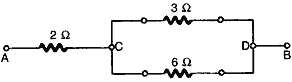The parallel combination of 3 Ω and 6 Ω resistors gives a value of:
1/R = 1/3 + 1/6 = 3/6
or          R = 2 Ω.
Now, this 2 Ω and the 2 Ω between A and C are in series. Therefore, equivalent resistance is
Re = 2 + 2 = 4 Ω.

(b)
To get a resistance of 1 Ω from three given resistors of resistance 2 Ω, 3 Ω, 6 Ω, we should connect all of them in parallel.

Hence, the equivalent resistance is given by
1/Re = 1/2 + 1/3 + 1/6 = 6/6 = 1
or Re = 1 Ω

#### Question: What is (a) the highest (b) the lowest total resistance that can be secured by combination of four coils of resistances 4 Ω, 8 Ω, 12 Ω, 24 Ω?

Answer: (a) The highest resistance is secured when all the four coils are connected in series. In that case,
R = 4 + 8 + 12 + 24 = 48 Ω.
(b) The lowest resistance is secured when all the four coils are connected in series. In that case,

1/R = 1/4 + 1/8 + 1/12 + 1/24 = 12/24
or      R = 0.5 Ω.

#### Question: Why does the cord of an electric heater not glow while the heating element does?

Answer: The cord of an electric heater is made up of metallic wire such as copper or aluminum which has low resistance while the heating element is made up of an alloy which has more resistance than its constituent metals. Also heat produced ‘H’ is
H = I2Rt
Thus, for the same current H oc R, so for more resistance, more heat is produced by heating element and it glows.

#### Question: Compute the heat generated while transferring 96000 C of charge in one hour through a potential difference of 50 V.

Answer: Given, Q = 96000 C,
t = 1 h = 60 × 60 = 3600 s, V = 50 V
Now, heat produced,
W = Q V = 96000 × 50 = 4.8 × 10J

#### Question: An electric iron of resistance 20 Q takes a current of 5 A. Calculate the heat developed in 30 s.

Answer: Given R = 20 Ω, I = 5 A, t = 30 s
H = I2Rt = (5)2 x 20 x 30 = 15000 J = 1.5 x 104 J

#### Question: What determines the rate at which energy is delivered by a current?

Answer: Electric power determines the rate at which energy is delivered by a current.

#### Question: An electric motor takes 5 A from a 220 V line. Determine the power of the motor and the energy consumed in 2 h.

Given I = 5 A, V = 220 V, t = 2 h Power,
p = VI = 220 x 5 = 1100 W
Energy consumed = Vlt = Pt
= 1100 x 2 = 2200 Wh

#### Question: How is a voltmeter connected in the circuit to measure the potential difference between two points?

Answer: A voltmeter is connected in parallel across any two points in a circuit to measure the potential difference between them with its +ve terminal to the point at higher potential and -ve terminal to the point at lower potential of the source.

#### Question: A copper wire has a diameter 0.5 mm and resistivity of 1.6 X 10-8 Ωm. What will be the length of this wire to make its resistance 10 Ω? How much does the resistance change if the diameter is doubled?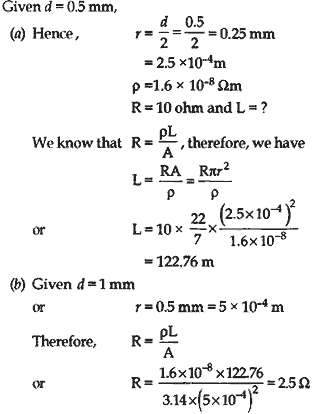####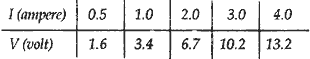Plot a graph between V and I and calculate the resistance of that resistor.

Answer: The V-I graph is as shown below: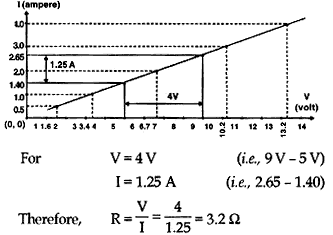#### Question: When a 12 V battery is connected across an unknown resistor, there is a current of 2.5 mA in the circuit. Find the value of the resistance of the resistor.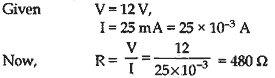#### Question: A battery of 9 V is connected in series with resistors of 0.2 Ω, 0.3 Ω, 0.4 Ω, 0.5 Ω and 12 Ω, respectively. How much current would flow through the 12 Ω resistor?

Answer: Since all the resistors are in series, equivalent resistance of the combination is
R = 0.2 + 0.3 + 0.4 + 0.5 + 12 = 13.4 Ω
Hence, current through the 12 Ω (since all the resistors are connected in series) resistor is
I = V/R = 9/13.4 = 0.67 A

## Sources of Energy: 10th Science Chapter 14

Class: 10th Class Subject: Science Chapter: Chapter 14: Sources of Energy Quiz: – Questions MCQs: …